Register
 Email: Passwd 验 证:换一张 Have you forgotten your password? Stay signed in Log In
 ALL Title Author Keyword Sponsors Type Publication date Submitted Time Subject Conference Name Source Categories KOS Subject Advisor ORCID Advanced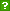ISCAS OpenIR  > 软件所图书馆  > 期刊论文
 Title: Multi-neighboring grids schemes for solving PDE eigen-problems Author: Sun JiaChang Keyword: PDE eigen-problem ; discrete Rayleigh quotient ; multi-neighboring grids schemes ; B-splines Source: SCIENCE CHINA-MATHEMATICS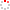Issued Date: 2013 Volume: 56, Issue:12, Pages:2677-2700 Indexed Type: SCI Department: Chinese Acad Sci, Inst Software, Sci Computat Lab, Beijing 100080, Peoples R China. Abstract: Instead of most existing postprocessing schemes, a new preprocessing approach, called multineighboring grids (MNG), is proposed for solving PDE eigen-problems on an existing grid G(Delta). The linear or multi-linear element, based on box-splines, are taken as the first stage (K1Uh)-U-h = lambda(1M1Uh)-M-h-U-h. In this paper, the j-th stage neighboring-grid scheme is defined as (KjUh)-U-h = lambda(jMjUh)-M-h-U-h, where K-j(h) := M-j-1(h) circle times K-1(h) and (MjUh)-U-h is to be found as a better mass distribution over the j-th stage neighboring-grid G(Delta), and K-j(h) can be seen as an expansion of K-1(h) on the j-th neighboring-grid with respect to the (j - 1)-th mass distribution M-j-1(h). It is shown that for an ODE model eigen-problem, the j-th stage scheme with 2j-th order B-spline basis can reach 2j-th order accuracy and even (2j + 2)-th order accuracy by perturbing the mass matrix. The argument can be extended to high dimensions with separable variable cases. For Laplace eigen-problems with some 2-D and 3-D structured uniform grids, some 2j-th order schemes are presented for j <= 3. English Abstract: Instead of most existing postprocessing schemes, a new preprocessing approach, called multineighboring grids (MNG), is proposed for solving PDE eigen-problems on an existing grid G(Delta). The linear or multi-linear element, based on box-splines, are taken as the first stage (K1Uh)-U-h = lambda(1M1Uh)-M-h-U-h. In this paper, the j-th stage neighboring-grid scheme is defined as (KjUh)-U-h = lambda(jMjUh)-M-h-U-h, where K-j(h) := M-j-1(h) circle times K-1(h) and (MjUh)-U-h is to be found as a better mass distribution over the j-th stage neighboring-grid G(Delta), and K-j(h) can be seen as an expansion of K-1(h) on the j-th neighboring-grid with respect to the (j - 1)-th mass distribution M-j-1(h). It is shown that for an ODE model eigen-problem, the j-th stage scheme with 2j-th order B-spline basis can reach 2j-th order accuracy and even (2j + 2)-th order accuracy by perturbing the mass matrix. The argument can be extended to high dimensions with separable variable cases. For Laplace eigen-problems with some 2-D and 3-D structured uniform grids, some 2j-th order schemes are presented for j <= 3. Language: 英语 WOS ID: WOS:000328279100015 Citation statistics: Content Type: 期刊论文 URI: http://ir.iscas.ac.cn/handle/311060/16897 Appears in Collections: 软件所图书馆_期刊论文

 Files in This Item:

There are no files associated with this item.

 Recommended Citation: Sun JiaChang. Multi-neighboring grids schemes for solving PDE eigen-problems[J]. SCIENCE CHINA-MATHEMATICS,2013-01-01,56(12):2677-2700.
 Service Recommend this item Sava as my favorate item Show this item's statistics Export Endnote File Google Scholar Similar articles in Google Scholar [Sun JiaChang]'s Articles CSDL cross search Similar articles in CSDL Cross Search [Sun JiaChang]‘s Articles Related Copyright Policies Null Social Bookmarking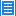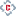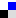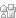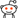您对该条目有什么异议，请填写以下表单，管理员会尽快联系您。 内 容： Email： * 单位： 验证码：刷新
 您在IR的使用过程中有什么好的想法或者建议可以反馈给我们。 标 题： * 内 容： Email： * 验证码：刷新

Items in IR are protected by copyright, with all rights reserved, unless otherwise indicated.Copyright © 2007-2019  中国科学院软件研究所 - Feedback Powered by CSpace